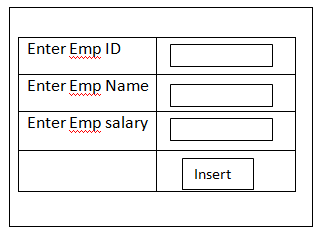# Display data using Razor syntax

21 September, 2018

Ratings

## Example to Display data of a table using Razor syntax

Create  a new view page  using with Razor engine

## ↓

Write the following code

```@ using system Data;
@using system Data.Sqlclient;
@ { layout = null ;
Sql connection  con = new sql connection (“”);
String s= “select * from emp details “;
Data set ds = new data set ();
Da.fill (ds,’x’);
}```
```<! Doctype html>
<html>
<title> Sample 1 </title>
<body>
<h2>Satya tech </h2>
<div>
<form action = “” Method = “Post”>
<table border = “2” align = ‘center’>
<tr>
<th>EMP id </th> <th> E name </th> <th> E sal </th>
</tr>
@ foreach (Data row R in ds. Tables . Rows)
{
<tr>
<td>@ R</td> <td>@ R</td><td>@ R</td>
</tr>
</table>
</div>
</body>
</html>```

Create new view page with the name (Samples)

`Learn the core features of ASP.NET Training and become master with our expertise tutorials.`Write the following code

```@using system. Data;
@using system. Data. Sqlclient;
@ { layout = null;
Var r = “”;
If (is post)
{
String s = “insert in to EMP Values  (”+ Request [“txt EMP id”] + “,”+Request [“txt E name”] “+”Request [txt E Sal]” +);
SQL connection = new sql connection (“”);
SQL command cmd = new dql command (s, con)
Con.open ();
Int i = cmd. Execute non query ();
Con. Close ();
R = I +”Record s inserted”
}
}
<body>
<h2>satya  technologies </h2>
<div>
<form action = ‘’ method = ‘post’>
<table border = ‘2’ align = ‘center’>
<tr>
<td>Enter EMP id </td> <td> <input type = ‘text’ name = ‘txt emp id’> </td>
</tr>
<tr>
<td>Enter E name </td> <td> <input type = ‘text’ name = ‘txt e name’> </td>
</tr>
<tr>
<td>Enter EMP Salary </td> <td> <input type = ‘text’ name = ‘txt emp  salary’> </td>
</tr>
<tr>
<td> @ R </td>  <td> <input type  = “submit ”  name = ‘B1 value = ‘’Insert’/>  </td>
</tr>
</table>
<tr>
<td>Enter Emp salary </td> <td> <Input type= “test” name= “txt emp sal”> </td>
</tr>
<td> @</td><td><input type = “submit”name =”Bi”,value =”insert”></td>
</tr>
</table>```

For indepth understanding click on